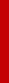#### Programs:

"Numerical solution of dynamic equilibrium models under Poisson uncertainty" (with Timo Trimborn), Journal of Economic Dynamics and Control 37 (2013): 2602-2622.  [Matlab implementation]

 Notes: The Matlab files illustrate how to apply the Waveform Relaxation algorithm to solve a neoclassical model with disasters. They also reproduce Figures 1 - 2 from Posch and Trimborn (2013). Choose the directory "Waveform" as current Matlab directory. execute "rbc.m" to start the calculations. The Figures show the policy function, the deviation from the last iteration, and absulte and relative errors (if available). to modify the model open "rbc.m" (main file), "funcODE.m" (set of differential equations), and "findss.m" (steady state conditions).